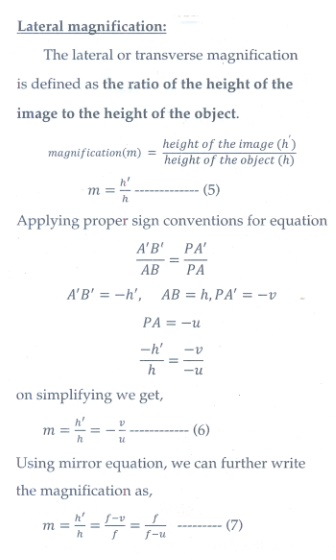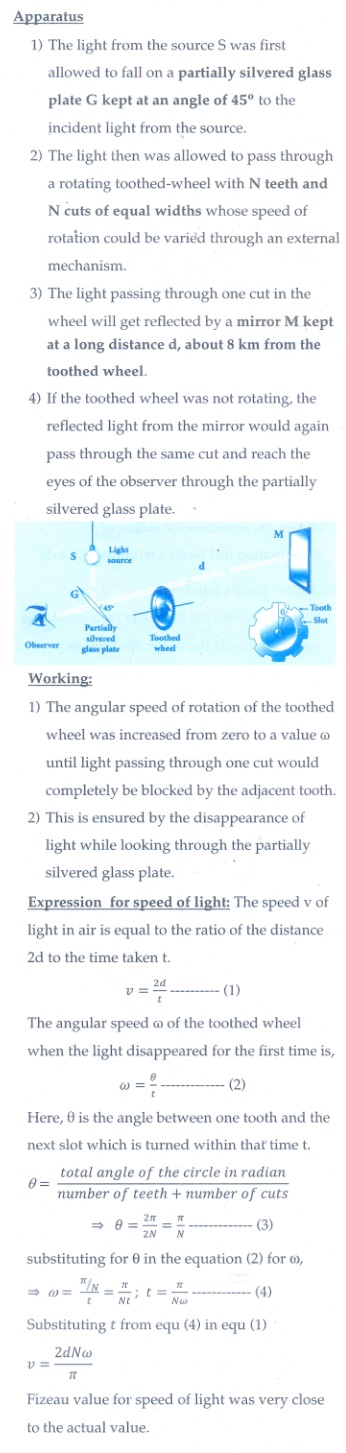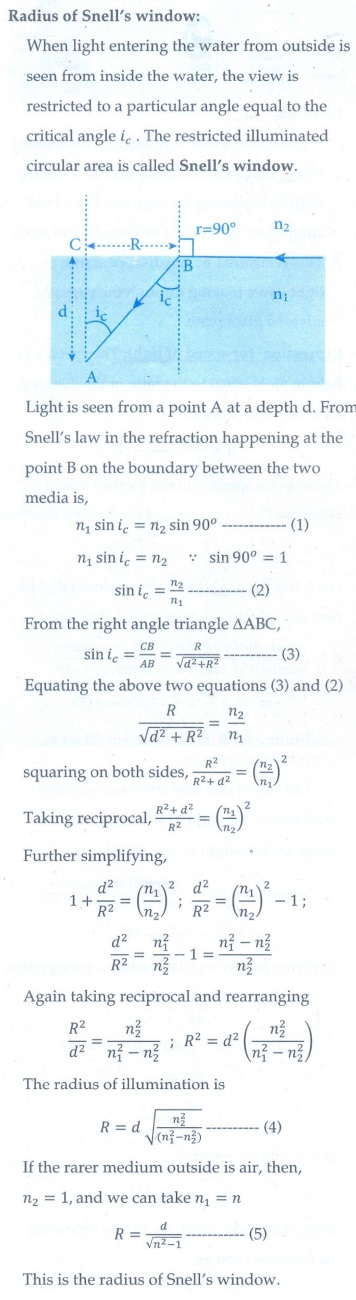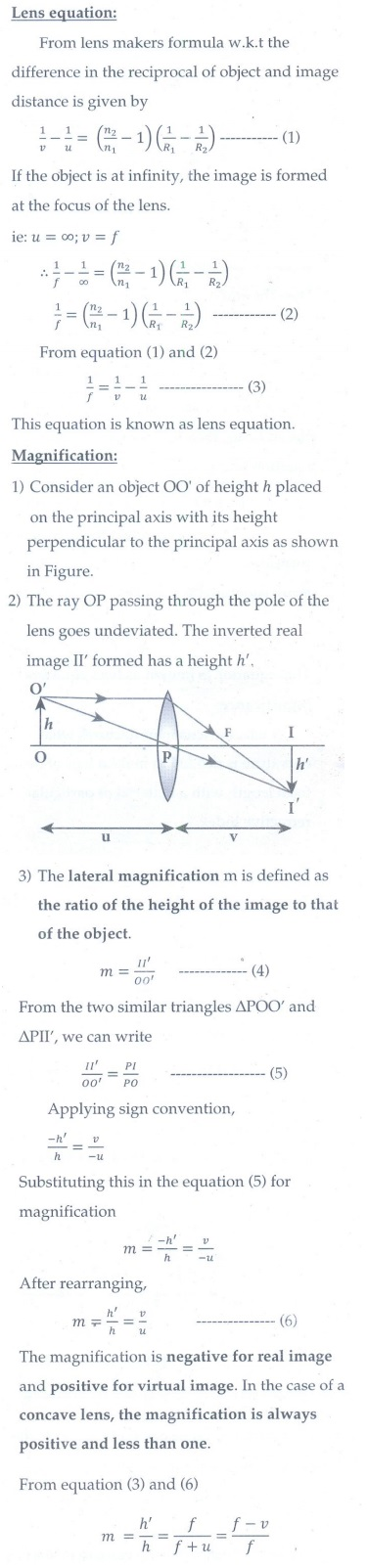Home | | Physics 12th Std | Long Answer Questions

## Chapter: 12th Physics : UNIT 6 : Ray Optics

Physics : Ray Optics: Book Back Questions, Answers, Solutions , Long Answer Questions

1. Derive the mirror equation and the equation for lateral magnification.2. Describe the Fizeau’s method to determine speed of light.3. Obtain the equation for radius of illumination (or) Snell’s window.4. Derive the equation for acceptance angle and numerical aperture, of optical fiber.5. Obtain the equation for lateral displacement of light passing through a glass slab.6. Derive the equation for refraction at single spherical surface.7. Obtain lens maker’s formula and mention its significance.8. Derive the equation for thin lens and obtain its magnification.9. Derive the equation for angle of deviation produced by a prism and thus obtain the equation for refractive index of material of the prism.10. What is dispersion? Obtain the equation for dispersive power of a medium.11. Derive the equation for effective focal length for lenses in out of contact.

Tags : Ray Optics | Physics , 12th Physics : UNIT 6 : Ray Optics
Study Material, Lecturing Notes, Assignment, Reference, Wiki description explanation, brief detail
12th Physics : UNIT 6 : Ray Optics : Long Answer Questions | Ray Optics | Physics